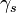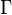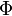# Calculation of sun position

This page describes several different approaches to calculation of sun position that are available using ASCEND. The first is a pure-ASCEND implementation of the algorithm from the book by Duffie & Beckman. Several other more accurate sun position algorithms such as the Grena algorithm are described further on, and work is underway to implement these algorithms as external relations and to tie them to our data reader for weather data.

## Duffie & Beckman algorithm

The ASCEND model library contains a file models/johnpye/sunpos_db.a4c that can be loaded in ASCEND and used to calculate the position of the sun at any location on earth and at any time of day or day of the year. It uses the method of Duffie and Beckman, which is accurate to the extent required for solar thermal energy calculations, but probably unsuitable for use in astronomy. Some more recent and more accurate correlations are given in the links at the bottom of this page.

The following is a code snippet, taken from the models/johnpye/sunpos_db.a4c file, that solves Example 1.6.1 from that book. It can be run and solved using ASCEND with the command ascend johnpye/sunpos_db.a4c.

(*
on a surface at 10:30 AM solar time on February 13, if the surface is
tilted 45 from the horizontal and pointed 15 degrees west of south.
*)
MODEL example_1_6_1 REFINES sunpos;
METHODS

METHOD specify;
RUN sunpos_db::specify;
FREE t;
FIX t_solar;
END specify;
METHOD values;
RUN sunpos_db::values;
t_solar := 43{d} + 10{h} + 30{min};
beta := 45 {deg};
gamma := 15 {deg};
L_st := -90{deg}; (* USA Central time*)
L_loc := -89.4{deg};
phi := +43{deg};
END values;
METHOD self_test;
ASSERT abs(theta-35.0{deg}) < 0.15{deg};
ASSERT abs(delta-(-13.80{deg})) < 0.02{deg};
END self_test;
END example_1_6_1;


Note that Duffie & Beckman define solar azimuth$\gamma_s$ (gamma_s in our model) as measured westward from south (i.e., 0°=south, 90°=west, -90°=east).

It is intended that this sun position calculation would be linked together with the experimental Data reader component to allow solar energy engineering problems to be solved over the course of days, weeks or years, along the lines of the TRNSYS simulation tool.

## Grena algorithm

Code to implement the Grena algorithm is given in models/johnpye/grena. This algorithm is most accurate (±0.0027°) in the range 2003-2023, although it will return results for a much wider range of dates. Be particularly careful if using it for dates before 2000, as it will make leap-day errors (we could easily modify Grena's code to correct that but as yet have not done so).

Grena defines solar azimuth$\Gamma$ as measured westward from south.

A sample model showing use of the Grena algorithm is models/johnpye/grena/sunpos_test.a4c.

## NREL Sun Position Algorithm

Error creating thumbnail: File missing
Solar analemma at Greenwich, calculated using the study function with the model models/johnpye/nrel/sunpos_nrel.a4c calculated every noon in the year 2000.
The NREL sun position code of Reda & Andreas is an implementation of an algorithm by Meeus and calculates accurate sun azimuth and zenith angles from 2000 BC to AD 6000, with claimed accuracy of ±0.0003° for that period. It makes use of atmospheric pressure, temperature and elevation to aid in accurate calculation including atmospheric refraction effects. It is more computationally expensive than other other (Grena, Duffie & Beckman) approaches (but not particularly expensive in comparison with calculation of accurate thermodynamic properties).

Connection of the NREL SPA to ASCEND is in progress. The code is at models/johnpye/nrel.

Reda and Andreas define solar azimuth$\Gamma$ ('topocentric astronomers azimuth angle') as measured westward from south. Note that they also define the 'topocentric azimuth angle'$\Phi$ as measured eastward from north, but since this conflicts with both Grena and Duffie & Beckman, we will adopt the$\Gamma$ measure.

## PSA sun position code

The sun position code from the Plataforma Solar de Almería (PSA) by Blanco et al is accurate to ±0.0083° for years 1999 to 2015.

There are Python bindings to the PSA sun position code via the package sunpos available from PyPI. Install it using pip install sunpos.

We have not yet implemented the PSA code in ASCEND, but plan to do so for comparison purposes.